## Friday, 19 November 2010

### Repeat of an Isoperimetric Discovery

It's that time of year again, so I want to repeat this to explain some ideas I laid out in Calculus today...

Recently we have been doing maxima/minima applications in calculus. By the time they get her most of them know that when they maximize the area of a rectangle with perimeter constraints, they get a square. In Pre-calc we do some basic problems with 2 adjacent pens, a single pen against a wall or river, and all the similar cases. I have written about the generalizations of some of these here, and here. The problem of two adjacent congruent pens is (or should be) easy for a calculus or precalc student.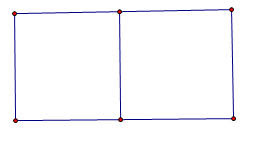It turns out that the maximum occurs when the total width is one-fourth the perimeter and the height is one-sixth the perimeter, for a maximum area of one twenty-fourth of the perimeter value (in square units, of course). If you extend that to even more congruent rectangular pens placed end to end it turns out that the width stays the same?

If you extend this, as many teachers do, to a shape like this one, it starts to get interesting to the more observant student.The height is now one-eighth of the total , and the width is one-sixth of the perimeter. Even slow students can figure out that the area of the total turns out to be one forty-eighth of the value of the perimeter. Some will even generalize the area of an array of mxn congruent pens to be the perimeter squared divided by [4(m+1)(n+1))].... but it seems to take forever for some one to finally realize that in all these problems, whether there was one rectangle, or one with one side adjacent to a river or barn, or rows of rectangles... there is one invariant...

Theorem one for rectangular arrays of congruent rectangular pens "The amount of fence going right to left is the same as the amount going up and down." If you want to maximize a rectangular array with five rows of three pens in each row, we will need 24 lines of fence, four going vertically, six going horizontally. Use half the fencing to make the four vertical fences, and the other half to make the other six going horizontally. (Prove..... dear reader) ..

I always thought this fifty-fifty use of fencing was a cute mathematical "teacher trick" but I have recently come to believe there is something deeper at work. I played around recently with this problem but loosened the constraints on the pens to be just equal area rectangles rather than congruent. This offered some nice areas to explore. Which would be better, three congruent pens in a row, or two pens with a third beneath... like so.The three adjacent pens turn out to have a max area when the width was p/4 (where p is the perimeter) and the height was p/8, giving a maximum area of p2/32... and as before, that mean that half the perimeter was used for the vertical sections, and half for the horizontal sections..and for the three non-congruent sections with equal area the width was p/6, and the length was 3P/16, giving a max area of....wait, that's also p2/32. (Ok, I hadn't expected that)... but when I checked the dimensions, it turns out that the horizontal sections use up exactly half the perimeter, and the vertical sections the other half in both cases.... hmmm...

Ok, but every kid knows that the area is maximized when the rectangle is closest to a square... so what if we just put three squares arranged as shown below and didn't require that the whole assembly forms a rectangle... I thought that would probably be even more area..It turns out that it isn't, The equal distribution of left and right still works out, but the total area is only 3p2/100 or about 96% of the other shapes. (ok, I wasn't ready for that)...

So, I decided to move up to five pens... I passed over four absolutely sure that the division into four equal squares in a two by two array would be the most efficient, but five offered several variations, five in a row; two rows of two with a single in the third row, or three in one row and two in another... The five congruent squares in a row gave a max area of p2/48, but the set up with three pens in one row and two in another gave a slightly larger area of 5p2/216 or p2/43.2. The 2,2,1 set up (shown below) gave not quite the same area..This set up gave a max area of p2/44.8.

From all of this I have a formed a totally unproven conjecture about the maximum area when n equal area rectangular pens are set out in a rectangular boundary with a given amount of fencing.
The Theorem One above for congruent rectangles seems to be a stronger property than I suspected, and is applicable to all equal area pens in a rectangular boundary, And the conjecture is that when the number of pens, n, is between x2-x and x2+x, the maximum area will be found when there are x-rows of pens and all of the pens have either x-1, x, or x+1 pens in the row.

The number of pens in any row will never differ by more than one from any other row. For values of n that are perfect squares or pronic (product of consecutive integers), the number of pens in each row will be the same. When n > x2 there will n-x2 rows that have x+1 pens, and the remaining rows will all have x pens. Similarly, if n is less than x2 there will be x2 - n rows that have x-1 pens and the remaining rows will have x pens each. As an example, if n= 23, then x=5 since 52-5 is less than 23 which is less than 52+5. And for 23 pens there will be five rows with x=five pens in three of them, and x-1=four pens in two of them.

I also have discovered that the theorem about equal lengths of fencing horizontally and vertically seems to be more important as a "rule of thumb" for maximizing than is equal sides. For example if five squares are set out as efficiently as possible (four in a square with one attached to a side), the area will be p2 / 45 with 8/15 of the perimeter going one way(say horizontally) and 7/15 going the other. If we change the squares to rectangles with each having a height of p/16 and a width of p/14 the area is p2/44.8.. an improvement of about 1/2 %....
Even if you go to six pens (where there is no lost perimeter enclosing the odd pen), putting two rows of three squares gave me an area of P2 / (48 1/3) but using the equal division of fencing gives the very slightly improved maximum of p2/48.

I can't explain why, but the equal division of fencing theorem is stronger than I first suspected, and if someone out there can shed more light on it than my feeble exploration, please do.

I haven't had time to expand the formula for the max area of all n when a rectangle is divided into congruent area rectangles, (not too difficult, I think).. but for square or pronic numbers of pens, it seems the max area will always be p2/(4 (x+1)(y+1)) where xy=n. As a limit as n approaches infinity, it seems the max area for rectangles divided into n equal area rectangles approaches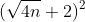## Thursday, 18 November 2010

### Plus or Minus InfinityCame across the problem above at the Math Central web page from Canada. I had written before about the values of iterated roots, but had not considered the case of what happens if they are joined by subtracting instead of adding. It turns out that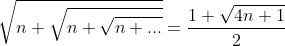. The derivation of this can be found in a short paper I wrote here.

By a similar approach we see that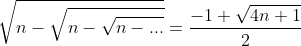making the problem above pretty simple as an aftermath of all this... but I wondered if there was an intuitive approach to justify this without the actual itereation results....nothing jumps out yet....

It also made me wonder about alternating signs...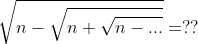.

Playing around with this I came up with a fourth degree equation to solve, with two positive roots...Yikes, but one of them was a spurious solution created by a second squaring of the equation. When I worked it out for n=2, I got a real surprise.. it led me back to the itereated sequence which started me on this exploration way back when...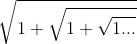... it turns out that the iteration of roots of two using alternating signs is deeply related to the iteration of roots of one using addition. I'll leave it to you to explore...

And their difference, if I have calculated correctly, is the iterated square roots of 3 using alternating signs.... Too much to explore,...... more later, but I just figured out in class today (I hope) that the series with alternating signs will equal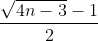and produces an integer value when the value of n is =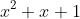for integer x.

## Wednesday, 10 November 2010

### Centroids of Inscribable Polygons

The term centroid, or center of gravity, of a triangle usually refers to the center of gravity obtained by treating the vertices as point masses with a mass of one unit. This leads to the centroid noramally found in geometry by intersecting the three medians. If we have points (xA,yA), (xB,yB), and (xC,yC) it is well known, and easily derived, that the centroid would be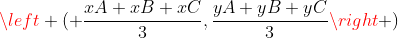.

There are other types of centroids, and one of them has an interesting relation to the class of polygons mentioned in the title. Another center of gravity can be found if we use the boundary of the polygon and assume it is made up of uniform rods. For a triangle, this produces a center of gravity that is at the intersection of the angle bisectors of the medial triangle (the triangle with vertices at the midpoints of the original triangle). This point is also called the Spieker Center of the triangle. To distinguish these two types of centroids, I will call the center of gravity of the vertices Gp (for point) and the center of gravity of the boundary Gb.

Some interesting sidebars about the angle bisectors of the medial triangle before I continue about centers of gravity.... The angle bisectors of the medial triangle are always parallel to the angle bisector of the opposite vertex. Also, these lines are each a "cleaver" of the triangle; a term created by Dov Avishalom in a Mathematics Magazine article for a line that passes through the midpoint of one side and divides the perimeter of the triangle into two equal halves.

I recently came across a paper by Tom Apostle and Mamikon A. Mnatsakanian that pointed out that in any triangle the two centroids and the incenter were always collinear. They also show that the ratio of the distances of the two centroids from the incenter is always in a ratio of 3/2. The ratio Gb/ Gp= 3/2.

Then in a really nice generalization, they showed that the same property applies to any inscribable polygon, one in which each edge is tangent to a common circle, such as the quadrilateral below. They use the clever term "circumgons" in the paper for such shapes. This is really a nice term since "inscribable" and "circumscribable" are used both to describe the circle inscribed in a polygon, or the polygon circumscribed about the circle... even I get confused.. Since it is often much easier to find Gp and the incenter of inscribable polygons, this provides a clever way to find Gb.

Students may be interested to know that Archimedes used the formula Area= 1/2 P r for the area of a circle, where P is the perimeter (circumference). This is, of course, simply and alternate form of the formula they learned in grade school. They may be even more surprised to find that the Archmedian formula gives the area of any inscribable polygon if P is the perimeter of the polygon, and r is the radius of the inscribed circle. The proof is very easy, and is often provided in high school textbooks for the limited case of regular polygons which would always be inscribable. The image below provides non-subtle clues to the proof.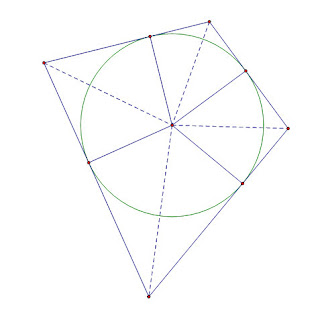## Friday, 5 November 2010

### Dollar Bill TrigonometryJust in time for my introduction to trig identities, Kate Nowak at f(t) reminded me of a beautiful geometric property of dollar bills.

Kate shows how to fold the dollar step by step, transforming it along the way from a rectangle to an isosceles trapezoid, then to a rhombus, and finally an equilateral triangle. And you can tease out a pretty decent regular tetrahedron with no overlap.

All this goes to show that the ratio of the height and width of a dollar bill is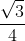...
But that is an important number.. that is the area of an equilateral triangle with unit sides. So if you made an equilateral triangle with all three sides the width of a dollar bill, then it would have the same area as the dollar bill... And the tetrahedron made from the dollar fold has the same surface area as well.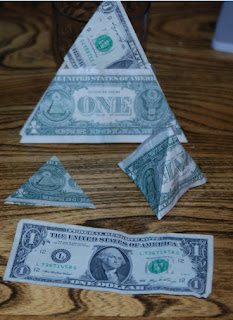The first two folds are pretty interesting for even a more advanced class... they form a 30-60-90 triangle, and it might be kind of nice to challenge students to explain WHY??? If it is not a 30 degree right triangle, then the whole equilateral triangle thing is a mess. The key is actually in recognizing that we have done what students/teachers may have heard is impossible, we have trisected an angle.

I was first introduced to this little dollar folding trick by a fourteen year old in my algebra II class some years ago..."Hey, Mr Ballew, bet you can't fold a dollar into an equilateral triangle." After I figured it out... (it took me a while).. I challenged him to explain why the first fold made a 30 degree right triangle. I can't tell you how proud it made me when seconds later he said, "Oh, it's because all three angles are equal."Quite clearly the folded portion is the same angle as the triangle where it would be "unfolded", so those two angles are congruent...and the third angle, well the length of the hypotenuse is one dollar bill height, and the opposite side is 1/2 a dollar bill height, so it is a 30 degree angle...which means the other two congruent angles must each be 30 degrees...

SPOOKY fun with folding money

Ok, it works with a fifty or a hundred bill too, all US bills are the same dimension (not true in most countries)...but hey, I'm on a teacher's pay, so I use ones...

Truth in Blogging Details.... AS wonderful as I would like this to be..the dollar seems NOT to be in a ratio ofexactly... Most places I have searched say the US dollar bill is 6.6294cm (2.61") wide, by 15.5956cm (6.14") long.. that is a ratio of 0.425081433... the square root of three divided by four turns out to be about 0.433012702... a difference between the two ratios of .008....

## Thursday, 4 November 2010

### Complex and Other Conjugates

We had covered the Fundamental Theorem of Algebra in pre-calc and after a few days of trying to make sure they understood how to decide how many real and non-real solutions there were to a real valued polynomials and that they could be factored over linear and quadratic factors , I was trying to get them to see how easy it was to work back and forth from the quadratic polynomial and the complex zeros. Finally I told them, "It's almost like the Pythagorian Theorem. a2+ b2 = c " [They will later learn that this is really just the product of two equal pythagorean results for each of the conjugates, but not yet]

I showed them that the complex solutions always came in conjugate pairs, a + bi and a - bi... and that if they knew the zeros, they could create a quadratic which had those solutions. It seems that students are no longer exposed to what I call the sum and product property of quadratic zeros... that if the zeros of a quadratic are r and s, a quadratic with those zeros is given by x2 - (r+s)x + (rs)... (If you knew the zeros were at x=3 and x= -1, then a quadratic with those zeros would be x2 - (3-1)x + (3*-1) or x2 - (2)x -3).

They had to get it somewhere if they were ever taught to factor, but it never seems to find purchase in their mathematical memory. I pointed out that for complex conjugates, r+s was real since the complex +bi and - bi eliminated each other, and so r+s was just twice the real coefficient of either conjugate. I connected this to the graph by showing that this "a" in the a+bi was the axis of symmetry of the graph. For example a quadratic with complex zeros at 3+2i and 3-2i will have a linear term of -(3+3)x [assuming we use the easiest case with A=1]. This also means that the of the quadratic will have its vertex at a point with an x value of 3.

It is also easy to see the constant term of the quadratic. If you multiply (a+bi)(a-bi) the distribution gives a2 +abi - abi - b2i2... As with the sum, the two imaginary terms will cancel out, and since i2=-1, we see that the product of the two complex conjugates will always be a2 + b2. So for the example 3+2i and 3-2i, the product of the conjugates is just 9+4=13. Putting this in as the constant term, we see that the quadratic x2 - 6x + 13 has zeros at 3+2i and 3-2i.

This gives another way to solve a quadratic equation with complex roots. If we have a function x2 - B x + C that we know has complex solutions, we know that the real coefficient, a, of the conjugate pair will be -B/2 (shades of the quadratic formula)... and we know that a2 + b2 = C, so we can figure out b pretty simply. If we had a quadratic such as x2 - 8 x + 25 we would quickly see that the real coefficient is -(-8)/2 = 4... and know that 42 + b2 = 25, so b must be 3, and the two solutions are at 4 +/- 3i. I finally admit to the students that this is just a variant of the quadratic formula, and sooner or later someone will ask if it is not possible to do the same thing with a quadratic with real zeros... I let them suggest a quadratic, and only press for the use of an even B in the linear coefficient for ease of explanation. For example with x2 - 6 x + 5 (ok, I picked an easy one).. we would see that the "a" part was three again... but now we have 32 + b^2 = 5.

If we remember that b was the imaginary coefficient, it makes sense that the b^2 would be negative if the zeros were real... Kids translate that into, "just subtract instead of add, and take the square root of that for the "b" value. So 9 - 4 = 5, and the square root of 4 is two. Our real solutions are 3 +/- 2; or 5 and 1.
At this point I show them that with real rational coefficients, not only do non-real zeros come in conjugate pairs, but so do the real irrational zeros. If we had x2 - 8 x + 10; the solutions of 4 +/- sqrt(16-10) would be the conjugate irrational solutions.

If we graph y=x2 - 6x + 13, we notice that not only is the vertex at x=3, but its y coordinate is at 4... the square of 2, which was the b part of our complex conjugates... It seems that functions with complex solutions a +/- bi, the vertex is at (a, b2). This also works for real valued zeros if we with the a +/- b approach above. The vertex of x2 -6x + 5 would be at (3, - 22).

I'm not sure I would try to drag a really weak class throuh all this, but it often helps bright kids see how interconnected the zeros, factors, and graphs of all quadratics are, not just the complex numbers.

## Tuesday, 2 November 2010

### A Catalog of Quadratic Equations

It is the time of year when I try to enchant my Pre-calc students with the beauty of complex solutions to polynomials, and particularly quadratics.. A good time to remind them of a beautiful result created by a high school student.....

-----------------------------------
The Mandelbrot set can be thought of as a catalog of closed Julia Sets over the complex plain. Each point on the plain representing a complex number, and the coloring representing whether the Julia set for that point had a closed or open orbit.

The equation y= 1/4 x2 on the coordinate plain can, in similar fashion, serve as a catalog of all possible quadratic equations. The method was discovered by a high school age British student by the name of Anthony Bayes around 1955 as reported in the Mathematical Gazette in September of that year by H. Gebert, the young man's teacher.

If every point (b,c) on the coordinate plain is representative of a quadratic equation x2+bx+c=0, then the set of all points in the cup of the parabola have only complex solutions [for example, the point (2,5) would represent the equation x2+2x+5=0 which has only complex roots]. Those points beneath the curve have two real distinct solutions, and the points on the curve specify equations which have a double root[ again, (2,1) would represent the perfect square trinomial x2+2x+1=0]. For any point on the curve, the solutions are -1/2 the x-coordinate. In the case of (2,1) the double root of x2+2x+1=0 is at -1/2 of 2 = -1.Any line tangent to the cuve passes only through points representing equations which share at least one solution. The line y=x-1 which is tanget to the curve at the point (2,1). Since the solutions for x2+2x+1=0 are both -1; all the points on this tangent represent quadratic equations which have x=-1 as one solution. As a case in point, (or a point in this case), (5,4) is on the tangent line, and the solutions of the quadratic equation x2+5x+4=0 are x=-1 and x=-4. If you drew the other tangent to the parabola passing through the point (5,4) it would contain the point (8,16) on the parabola representing the quadratic with a double root at x=-4, that is, the quadratic x2+8x+16=0.

This would allow a simple approximation method to find the solutions of any quadratic with reasonably small values using only a straightedge and a printed graph of the curve y=1/4 x2. Simply pick the point (b,c) corresponding to the values of the equation, and then lay a straight edge through the point and tangent to the parabola. The solutions would be -1/2 the x values of the points of tangency, as given above.